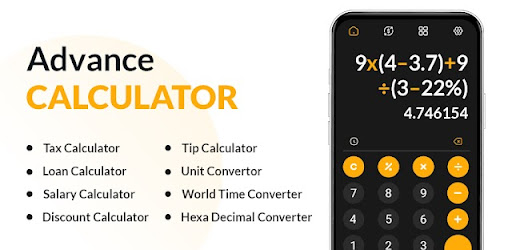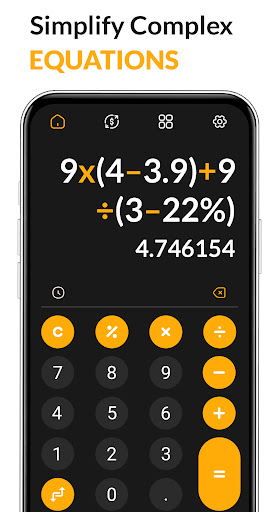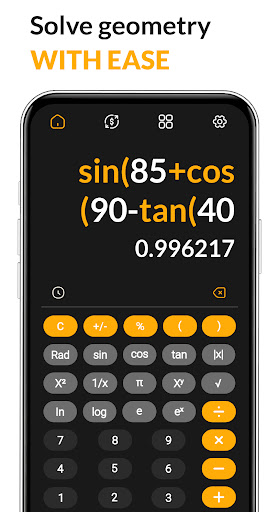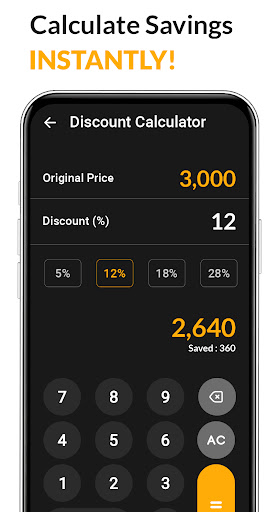# Calculator App - Scientific

by DevArt - VPN Proxy, Weather, Calculator & Recovery

All in one Scientific Calculator for math solutions, Calculator Plus for android

App Name Calculator App - Scientific DevArt - VPN Proxy, Weather, Calculator & Recovery Tools 6 MB 1.0.6 4.35 1,690 Download Download Calculator App - Scientific Android appThe Scientific Calculator and Simple Calculator app is a user friendly and intuitive calculator that enables users to perform basic calculations quickly and easily. Scientific Calculator features an easy to use interface with large, clearly labeled buttons and a display that shows the current calculation. Users can enter numbers using the number pad or use the built-in functions to perform more complex calculations. Additionally, the best Calculator plus app supports both portrait and landscape orientations for convenience.

Scientific Calculator:

A scientific calculator is a type of electronic calculator, usually but not always handheld, designed to calculate problems in science, engineering, and mathematics. Scientific Calculator free typically has a wide range of functions including trigonometric and statistical calculations and specialized functions for use in different fields. Most scientific calculators plus have an LCD display and usually have a two-line display that shows both the equation being entered and the result.

All in One Calculator:

The All in One Calculator App is a comprehensive calculator app that provides users with a wide range of functions and features. Scientific Calculator includes basic calculator functions such as addition, subtraction, multiplication, and division, as well as advanced scientific calculations such as trigonometry and logarithms.

Simple Calculator:

A Scientific calculator is a basic calculator that can perform basic arithmetic operations such as addition, subtraction, multiplication, and division. Simple Calculator+ is typically used for everyday calculations and does not have any advanced features.

GPA Calculator:

GPA Calculator helps students calculate their grade point average (GPA) from their course grades. Simply enter each course grade, along with its credit value, and Science Calculator will provide an overall GPA score for all courses taken.

Currency Calculator:

A Currency Converter is a tool used to convert one currency to another. Currency Calculator free can be used to compare prices of items in different currencies or to calculate the exchange rate between two currencies. With Scientific Calculator comprehensive coverage of global currencies, the Currency Converter App is a must have for anyone who frequently deals with multiple currencies.

World Time Calculator:

World Time Calculator allows you to quickly and easily calculate the current time in different locations worldwide. Simply enter the location and Smart Calculator displays the current local time.

Fuel Cost Calculator:

The Fuel cost calculator free helps you to estimate how much money you will spend on fuel for a given journey. Enter your starting and destination points, along with your vehicle's fuel efficiency, and best Calculator give you an estimate of how much it will cost to get there.

Age Calculator:

Calculator Plus can be used to quickly determine your age based on a given date of birth. Age Calculator free also provides a handy age comparison tool so that you can compare your age with someone else's.

Tax Calculator:

Best Scientific calculator is used to calculate taxes based on income, deductions, and other factors. Tax Calculator plus can help individuals and businesses determine how much they owe in taxes.

Salary Calculator:

Smart Calculator is used to calculate salary for a given job position or title. Salary Calculator takes into account the job's market rate, experience level, and other factors to determine an appropriate salary.

Loan Calculator:

Scientific Calculator is used to estimate loan payments, interest rates, and repayment periods. Loan free Calculator Plus can also provide an estimated total cost of the loan including interest over time.

BMI Calculator:

BMI free Science Calculator is used to calculate Body Mass Index (BMI). Smart Calculator takes into account height and weight of an individual to determine if they are within a healthy range or not.